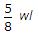# Civil Engineering - UPSC Civil Service Exam Questions

21.

In an aerial photo, the image of the top of a tower is found to be 10 cm from the centre of thephotograph. If the height of the tower is 100 m and the flying height of the aircraft is 1000 m above the average terrain height, then the height displacement of the image from the true position will be

 A. 10 mm B. 20 mm C. 50 mm D. 100 mm

Explanation:

No answer description available for this question. Let us discuss.

22.

Which of the following are the requirements for the design of a transition curve for a highway system ?
1. Rate of change of grade
2. Rate of change of radial acceleration
3. Rate of change of super elevation
4. Rate of change of curvature
Select the correct answer using the code given below :

 A. 1, 2 and 3 B. 2, 1 and 4 C. 1, 3 and 4 D. 2, 3 and 4

Explanation:

No answer description available for this question. Let us discuss.

23.

The void-pressure diagram is shown above. What is the coefficient of compressibility ?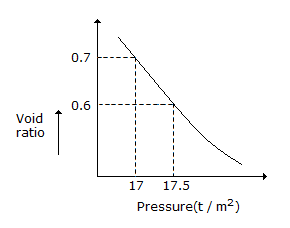A. 0.5 m2/t B. 0.73 m2/t C. 0.20 m2/t D. 0.25 m2/t

Explanation:

No answer description available for this question. Let us discuss.

24.

A propped cantilever of span 'L' is subjected to a concentrated load at mid-span. If Mp is plastic moment capacity of the beam, then the value of the collapse load will be

 A.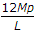B.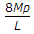C.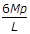D.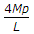Explanation:

No answer description available for this question. Let us discuss.

25.

A propped cantilever of span 'l' carries uniformly distributed load of 'w' per unit over its entire span. The value of prop react to keep the beam horizontal is

 A.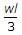B.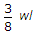C.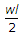D.